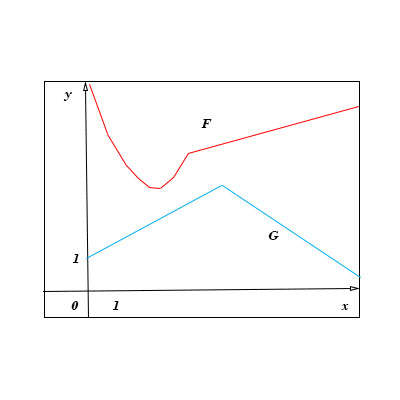# Let P(x) = F(x)G(x) and Q(x)=\frac{F(x)}{G(x)}, where F and G are the functions whose graphs are...

## Question:

Let {eq}P(x) = F(x)G(x) {/eq} and {eq}Q(x)=\frac{F(x)}{G(x)} {/eq}, where {eq}F {/eq} and {eq}G {/eq} are the functions whose graphs are shown.

a. Find {eq}F'(2) {/eq}

b. Find {eq}Q'(7) {/eq}## Derivatives of {eq}f(x)g(x) {/eq} and {eq}\frac{f(x)}{g(x)} {/eq}:

To differentiate functions that are the product or quotient of two different functions, we use given rules that have been derived for us. The multiplication rule gives us

{eq}\displaystyle \frac{d}{dx} f(x)g(x) = f'(x)g(x) + f(x)g'(x) {/eq}

and the Quotient Rule gives us

{eq}\displaystyle \frac{d}{dx} \frac{f(x)}{g(x)} = \frac{f'(x)g(x) - f(x)g'(x)}{\big(g(x) \big)^2} {/eq}

To find {eq}F'(2) {/eq} we would approximate the slope of a line tangent to {eq}F {/eq} at {eq}2 {/eq} by looking at the graph of {eq}F {/eq} . {eq}F(2) {/eq} appears to be the bottom of a parabola shaped curve So this slope would be zero, ie. {eq}F'(2)=0 {/eq}.

To determine {eq}Q'(7) {/eq} we need to use the Quotient Rule

{eq}\displaystyle Q'(7)= \frac{F'(7)G(7) - F(7)G'(7)}{\big( G(7) \big)^2} {/eq}.

By looking at the graph and approximating the values of {eq}F(7), F'(7), G(7), {/eq} and {eq}G'(7) {/eq}we get.

{eq}\displaystyle Q'(7) = \frac{(\frac{1}{2})(1) - (6)(-1)}{(1)^2} = \frac{13}{2} {/eq}